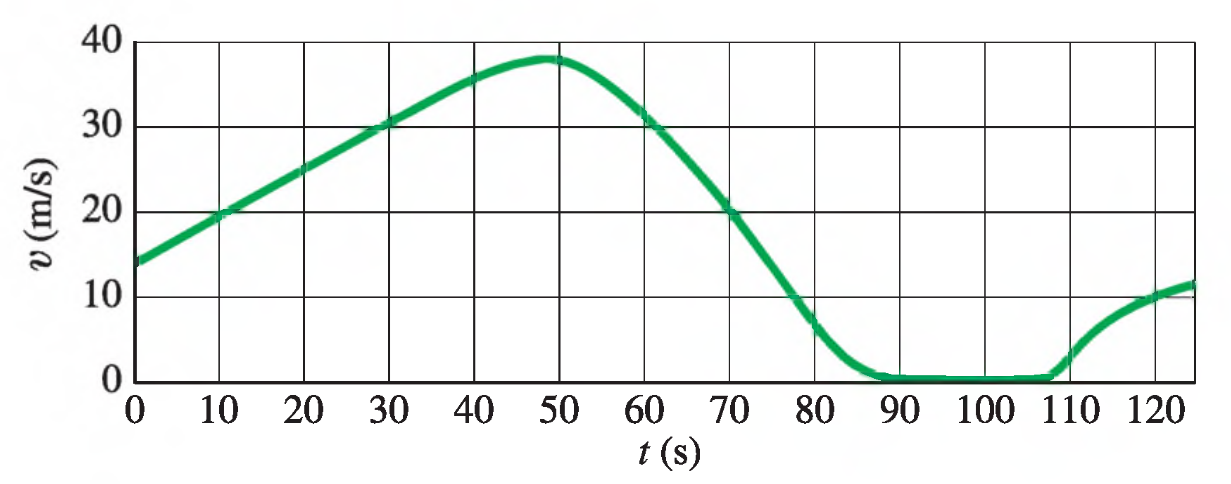# Problem: The figure shows the velocity of a train as a function of time.(a) At what time was its velocity greatest?(b) During what periods, if any, was the velocity constant?(c) During what periods, if any, was the acceleration constant?(d) When was the magnitude of the acceleration greatest?

###### FREE Expert Solution
94% (377 ratings)
###### FREE Expert Solution

We're looking at a velocity graph to determine some things about the velocity and acceleration of at train.

Anytime we're given a position, velocity, or acceleration graph to work with, a PVA diagram like the one below can help remind you of the relationships between the three functions.

$\mathbit{P}\begin{array}{c}{\mathbf{←}}\\ {\mathbf{\to }}\end{array}\underset{{\mathbit{s}}{\mathbit{l}}{\mathbit{o}}{\mathbit{p}}{\mathbit{e}}}{\overset{{\mathbit{a}}{\mathbit{r}}{\mathbit{e}}{\mathbit{a}}}{\mathbit{V}}}\begin{array}{c}{\mathbf{←}}\\ {\mathbf{\to }}\end{array}\mathbit{A}$

Moving left to right, we take the slope of the graph—the slope of a position graph is the velocity, and the slope of a velocity graph is the acceleration.

94% (377 ratings)###### Problem Details

The figure shows the velocity of a train as a function of time.
(a) At what time was its velocity greatest?
(b) During what periods, if any, was the velocity constant?
(c) During what periods, if any, was the acceleration constant?
(d) When was the magnitude of the acceleration greatest?